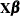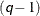# The SEVERITY Procedure

### SCALEMODEL Statement

• SCALEMODEL regression-effect-list </ scalemodel-options>;

The SCALEMODEL statement specifies regression effects. A regression effect is formed from one or more regressor variables according to effect construction rules. Each regression effect forms one element ofin the linear model structurethat affects the scale parameter of the distribution. The SCALEMODEL statement in conjunction with the CLASS statement supports a rich set of effects. Effects are specified by a special notation that uses regressor variable names and operators. There are two types of regressor variables: classification (or CLASS) variables and continuous variables. Classification variables can be either numeric or character and are specified in a CLASS statement. To include CLASS variables in regression effects, you must specify the CLASS statement so that it appears before the SCALEMODEL statement. A regressor variable that is not declared in the CLASS statement is assumed to be continuous. For more information about effect construction rules, see the section Specification and Parameterization of Model Effects.

All the regressor variables must be present in the input data set that you specify by using the DATA= option in the PROC SEVERITY statement. The scale parameter of each candidate distribution is linked to the linear predictorthat includes an intercept. If a distribution does not have a scale parameter, then a model based on that distribution is not estimated. If you specify more than one SCALEMODEL statement, then the first statement is used.

The regressor variables are expected to have nonmissing values. If any of the variables has a missing value in an observation, then a warning is written to the SAS log and that observation is ignored.

For more information about modeling regression effects, see the section Estimating Regression Effects.

You can specify the following scalemodel-options in the SCALEMODEL statement:

DFMIXTURE=method-name <(method-options)>

specifies the method for computing representative estimates of the cumulative distribution function (CDF) and the probability density function (PDF).

When you specify regression effects, the scale of the distribution depends on the values of the regressors. For a given distribution family, each observation in the input data set implies a different scaled version of the distribution. To compute estimates of CDF and PDF that are comparable across different distribution families, PROC SEVERITY needs to construct a single representative distribution from all such distributions. You can specify one of the following method-name values to specify the method that is used to construct the representative distribution. For more information about each of the methods, see the section CDF and PDF Estimates with Regression Effects.

FULL

specifies that the representative distribution be the mixture of N distributions such that each distribution has a scale value that is implied by each of the N observations that are used for estimation. This method is the slowest.

MEAN

specifies that the representative distribution be the one-point mixture of the distribution whose scale value is computed by using the mean of the N values of the linear predictor that are implied by the N observations that are used for estimation. If you do not specify the DFMIXTURE= option, then this method is used by default. This is also the fastest method.

QUANTILE <(K=q)>

specifies that the representative distribution be the mixture of a fixed number of distributions whose scale values are computed by using the quantiles from the sample of N values of the linear predictor that are implied by the N observations that are used for estimation.

You can use the K= option to specify the number of distributions in the mixture. If you specify K=, then the mixture containsdistributions such that each distribution has as its scale one of the-quantiles.

If you do not specify the K= option, then PROC SEVERITY uses the default of 2, which implies the use of a one-point mixture with a distribution whose scale value is the median of all scale values.

RANDOM <(random-method-options)>

specifies that the representative distribution be the mixture of a fixed number of distributions whose scale values are computed by using the values of the linear predictor that are implied by a randomly chosen subset of the set of all observations that are used for estimation. The same subset of observations is used for each distribution family.

You can specify the following random-method-options to specify how the subset is chosen:

K=r

specifies the number of distributions to include in the mixture. If you do not specify this option, then PROC SEVERITY uses the default of 15.

SEED=number

specifies the seed that is used to generate the uniform random sample of observation indices. If you do not specify this option, then PROC SEVERITY generates a seed internally that is based on the current value of the system clock.

OFFSET=offset-variable-name

specifies the name of the offset variable in the scale regression model. An offset variable is a regressor variable whose regression coefficient is known to be 1. For more information, see the section Offset Variable.